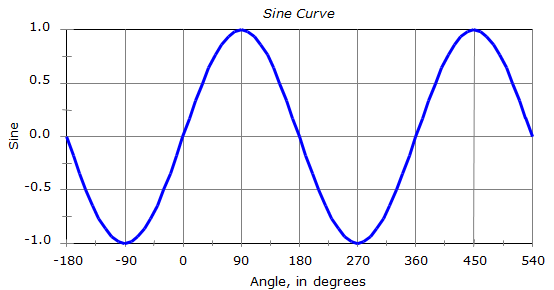## 2. Trigonometric Functions

### a. Sine; Law of Sines

The sine of any angle falls between -1.0 and +1.0. Refer to the sine curve plot in Figure B-4 to see how angles and their sines relate. This curve repeats itself every 360°;
so sin(-340°) = sin(20°) = sin(380°)...Figure B-4

Taking the arcsine (a.k.a. inverse sine, sin-1) of a number between -1.0 and +1.0 on a calculator will always return an angle between -90° and +90°.

For example:

Sin(101°) is 0.981627

Sin-1(0.866025) is 79°

We started with 101° and wound up with 79°.

Sly obersverse that we are, we notice that 101°+79° is 180°

Table B-1 show some other angles, their sine behavior, relationship between the beginnng and ending angles.

 Table B-1 α x=sin (α) sin-1(x) relationship 30° +0.50000 +30° α 150° +0.50000 +30° 180°-α 210° -0.50000 -30° 180°-α 330° -0.50000 -30° 360°+α

All of the angles in the third colum are between -90° and +90°. This is shown graphically in Figure B-5.Figure B-5 ArcSine Angle Range

A triangle may have an angle which is greater than 90° but if you are solving that angle using the Law of Sines, the computed angle will be less than 90°. Although no single angle in a triangle may exceed 180° the Law of Sines can result in two angles both less than 180°.

For example sin-1(0.5) can be 30° or 150° - both meet the sine condition but only one meets the triangle condition. A calculator will always return 30° even though the “true” angle is 150°. How do you know which is correct? It depends on the triangle.

#### (1) Example

In triangle ABC, a = 12.4', b = 8.7', and B = 36°40'.

Compute the remaining angles and side.

From the Law of SinesThere are two angles whose sine is +0.85112

A = 58°20' and A = (180°00' - 58°20') = 121°40', Figure B-6.Figure B-6 Multiple Angles with Same Sine

That means there are two different triangle solutions, Figure B-7Figure B-7 Two Possible Angle Values

Consider point C as the center of an arc of radius 8.7'.

This arc can intersect the remaining side at two points; A1 and A2.

Isolating the two triangles and solving their remaining components:

For A = 58°20'Figure B-8 First Solution

Angle conditionLaw of SinesFor A = 121°40'Figure B-9 Second Solution

Angle conditionLaw of Sines#### (2) Example 2

Compute the angle B in the following triangle:Figure B-10 Example 2

The missing angle can be computed two ways:

Law of SinesAngle conditionAs a result there are two "correct" answers fot the missing angle:

sin(108°) = sin(72°) = 0.95105652

Ans since neither exceeds 180°, either can be an angle of a triangle,

but only 108° fits both the Law of Sines and the Angle condition.

 Be careful when using the Law of Sines to solve for an unknown angle – there could be two possible answers only one of which will fit the particular triangle.

### b. Cosine; Law of Cosines

The cosine of any angle falls between -1.0 and +1.0. Refer to the cosine curve plot in Figure B-11 to see how angles and their cosines relate. This curve repeats itself every 360°; so cos(-340°) = cos(20°) = cos(380°)...

The cosine curve is identical to the sine curve except its phase differs by 90°.Figure B-11

Taking the arccos (a.k.a. inverse cosine, cos-1) of a number between +1.0 and -1.0 on a calculator will always return an angle between 0° and 180°; for example:

 Table B-2 α x=sin (α) sin-1(x) 60° +0.50000 +60° 300° +0.50000 +60° 120° -0.50000 +120° -240° -0.50000 +120°

All of the angles in the third colum are between 0° and +180°. This is shown graphically in Figure B-12.Figure B-12 ArcCosine Angle Range

Using the Law of Cosines will not cause an ambiguous solution as does the Law of Sines since any single angle in a triangle cannot exceed 180°.

To solve a triangle using the Law of Cosines you must have either three sides, or, two sides and an angle.

#### (1) Example 1

Compute the value of the angle R in the triangle below:Figure B-13 Example

From the Law of Cosines:The Law of Cosines returns only one legitimate value when solving triangles.

### c. Tangent

Unlike sine and cosine, the tangent of any angle not limited to the range of -1.0 to +1.0. As a matter of fact, the tangent range is ±∞ (infinity). You can see that the tangent function plot is not sinusoidal as are the sine and cosine plots. And unlike the other two it repeats itself every 180°;Figure B-14

The tangent curve is asymptotic at 90°, 270°, 450°, etc. Asymptotic means the curve gets close to, but never reaches, those values.
so tan(90°) = tan(270°) = ... = infinity

Try evaluating tan(90°) on your calculator; you’ll probably get an error statement of sorts. Then try tan(89.99999°); you should get a pretty big number. Why is that?

Recall that tan(α) = sin(α) / cos(α)

At 90°, cos(90°) = 0 so you get division by 0, hence tan(90°) = ∞.

There is a Law of Tangents, but we don't generally use it to solve triangles since the Laws of Sines or Cosines can be used instead. For extra credit, find and memorize it.